## Traveling Salesman Constants

N.B. A detailed on-line essay by S. Finch was the starting point for this entry.

Letbe the smallest Tour length forpoints in a-D Hypercube. Then there exists a smallest constant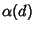such that for all optimal Tours in the Hypercube,(1)

and a constantsuch that for almost all optimal tours in the Hypercube,(2)

These constants satisfy the inequalities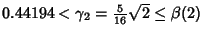(3)(4)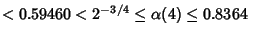(5)
(Fejes Tóth 1940, Verblunsky 1951, Few 1955, Beardwood et al. 1959), where(6)is the Gamma Function,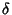is an expression involving Struve Functions and Neumann Functions,(7)

(Karloff 1989), and(8)

(Goddyn 1990). In the Limit,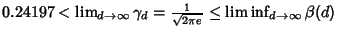(9)
and(10)

where(11)

andis the best Sphere Packing density in-D space (Goddyn 1990, Moran 1984, Kabatyanskii and Levenshtein 1978). Steele and Snyder (1989) proved that the limitexists.

Now consider the constant(12)

so(13)

The best current estimate is.

A certain self-avoiding Space-Filling Curve is an optimal Tour through a set ofpoints, wherecan be arbitrarily large. It has length(14)

whereis the length of the curve at theth iteration and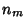is the point-set size (Moscato and Norman).

References

Beardwood, J.; Halton, J. H.; and Hammersley, J. M. The Shortest Path Through Many Points.'' Proc. Cambridge Phil. Soc. 55, 299-327, 1959.

Chartrand, G. The Salesman's Problem: An Introduction to Hamiltonian Graphs.'' §3.2 in Introductory Graph Theory. New York: Dover, pp. 67-76, 1985.

Fejes Tóth, L. Über einen geometrischen Satz.'' Math. Zeit. 46, 83-85, 1940.

Few, L. The Shortest Path and the Shortest Road ThroughPoints.'' Mathematika 2, 141-144, 1955.

Finch, S. Favorite Mathematical Constants.'' http://www.mathsoft.com/asolve/constant/sales/sales.html

Flood, M. The Travelling Salesman Problem.'' Operations Res. 4, 61-75, 1956.

Goddyn, L. A. Quantizers and the Worst Case Euclidean Traveling Salesman Problem.'' J. Combin. Th. Ser. B 50, 65-81, 1990.

Kabatyanskii, G. A. and Levenshtein, V. I. Bounds for Packing on a Sphere and in Space.'' Problems Inform. Transm. 14, 1-17, 1978.

Karloff, H. J. How Long Can a Euclidean Traveling Salesman Tour Be?'' SIAM J. Disc. Math. 2, 91-99, 1989.

Moran, S. On the Length of Optimal TSP Circuits in Sets of Bounded Diameter.'' J. Combin. Th. Ser. B 37, 113-141, 1984.

Moscato, P. Fractal Instances of the Traveling Salesman Constant.'' http://www.ing.unlp.edu.ar/cetad/mos/FRACTAL_TSP_home.html

Steele, J. M. and Snyder, T. L. Worst-Case Growth Rates of Some Classical Problems of Combinatorial Optimization.'' SIAM J. Comput. 18, 278-287, 1989.

Verblunsky, S. On the Shortest Path Through a Number of Points.'' Proc. Amer. Math. Soc. 2, 904-913, 1951.